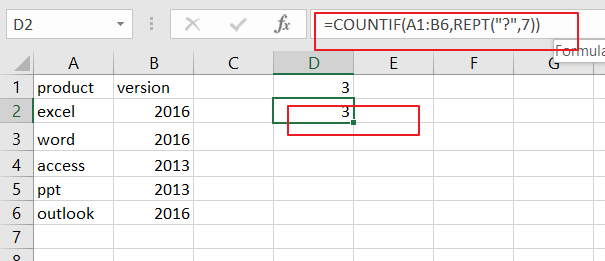# How to Count Number of Cells that Contain Exactly N Characters in Excel

This post will guide you how to count the number of cells that contain exactly N charcters within a range of cells using a formula in Excel 2013/2016.You can easily to count cells equal to a specific value or text string through COUNTIF function. But if there is an easy way to count cells that contain a certain length  in your data range.

## Count Number of Cells that Contain Exactly N Characters

If you want to count the number of cells that contain a certain length or a certain number of characters(7 characters) in range A1:B6, you can create a formula based on the COUNTIF function. Like this:

=COUNTIF(A1:B6,”???????”)

Or

=COUNTIF(A1:B6,REPT(“?”,7))

###You would see that the above formulas will count the number of cells in a given range A1:B6 which contain exactly 7 characters.

Note: A1:B6 is the range from which to count the cells that contain seven characters.

LET’S SEE THAT HOW THIS FORMULA WORKS:

This above formula will use the question mark “`?`” character to match one character. So if you wish to match seven characters, and you just need to add 7 characters into the formula.

The REPT function will return a text string made of exactly 7 question marks.

The COUNTIF function will count all the cells in the range that contain exactly 7 characters.

### Related Functions

• Excel REPT function
The Excel REPT function repeats a text string a specified number of times.The syntax of the REPT function is as below:= REPT  (text, number_times)…
• Excel COUNTIF function
The Excel COUNTIF function will count the number of cells in a range that meet a given criteria. This function can be used to count the different kinds of cells with number, date, text values, blank, non-blanks, or containing specific characters.etc.= COUNTIF (range, criteria)…